Journal of Water Resources and Ocean Science
Volume 5, Issue 6, December 2016, Pages: 114-121

Effect of Corrugated Rough Bed Channels on Sediment Transport Processes

Mohamed Gamal Abdalla

Irrigation and Hydraulics Dept., Faculty of Engineering, Mansoura University, Mansoura, Egypt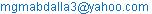Mohamed Gamal Abdalla. Effect of Corrugated Rough Bed Channels on Sediment Transport Processes. Journal of Water Resources and Ocean Science. Vol. 5, No. 6, 2016, pp. 114-121. doi: 10.11648/j.wros.20160506.16

Received: January 1, 2017; Accepted: January 13, 2017; Published: February 3, 2017

Abstract: In the present research, the effect rough corrugated bed channels on the sediment transport and of the flow passes is studied. Hypothetically, four scenarios of bed cases are tested. In the first case, using large eddy simulation, LES, an investigation of smooth bed is performed for ReT equals 395 (number of Reynolds calculated using the mean friction velocities at wall). The other three corrugated sinusoidal bed shape cases are assumed with different amplitudes and constant wavelengths. An eddy viscosity model which is adapted to wall is applied in this research; while the subgrid scale quantity is depend on the hypothesis gradient. A comparison between data of Cherukat et al.  obtained from Direct Numerical Simulation, DNS, and the data measured by Hudson et al.  with the corresponding computed flow is done. Then, wavy sinusoidal bed shape case is considered. From this study, it was found that the flow is affect strongly by the bed corrugations and the sediment transport is more sensitive for the larger heights of bed corrugations. Also, it is proved that the Rouse theory could be applied clearly in outer zone of the corrugated bed. It is explained generally in this paper that the profiles of turbulent Schmidt number are not affected by the height of corrugation.

Keywords: LES, Sediment Transport, Numerical Modeling, Rough Bed

Contents

1. Introduction

In many papers, the researchers study only the process of sediment transport in smooth bed channels. However, in the nature, the bed of any channel or river is rough and corrugated. The word roughness or corrugation means a lot, micrometers on pipes in laboratories such as the pipes from glass or millimeters in steel pipes or the concrete pipes or meters or more than that for rivers and alluvial channels etc. The main goal and the major interest in this paper are studying the transportations sediment rates through rough bed channels. As there is no more data in the flow process over rough bed channels, the low-Re k-ε turbulence model made by Heredia  for the flow process through rough bed channels is developed by providing LES data.

Many papers were published through the last years about the flow properties through rough bed channels without studying the sediment transport process. The first study was done by Nikuradse in  was to find the effect of bed corrugations and roughness on the flow and also, he studied the sensitivity of skin friction in pipes using roughness contains from sand. Then, Schlichting introduced the concept of the equivalent grain roughness of sand (ks).

Many papers about the topic such as Nakagawa and Hanratty , Nakagawa et al , Hudson et al. , Kuzan et al. , Einstein and Barbarossa , Grass , Lee , Miyake et al. , Orlandi et al. , , and , Leonardi et al. , Jimenez , Tseng and Ferziger , Ashraan et al. , Stoesser et al. , Bhaganagar et al. , Choi and Suzuki)  were published. In the aforementioned papers, the effect of wall roughness on the flow is well appear and known. Only there is few papers were published that studied experimentally on roughness channels the effect of roughness on the sediment transport rate, such as Boersma , or Cellino , and Graf and Cellino . Yoon and Kang  and Lely  studied numerically the sedimentation process over rough bed channels. The lack of the models of turbulences that gives an excellent agreement in the near bed zone that affected by the elements of roughness represents the greatest challenge in the problem of large scale sediment transportation process modeling. Heredia  provided a new two layer turbulence model based on current LES data for the flow over roughness bed. In his study, the new model overcomes the current models of two layers and could be applied for clear and sediment laden flow. It is also calibrated with the LES data and the turbulent behavior at the zone near the wall and the variations of theses quantities due to rough bed is reproduced. This model was validated successfully with DNS, direct numerical simulation, with free water surface case based on experimental results.

In this research, the effect of different bed shapes such as sinusoidal, wavy on the flow will be investigated using LES. Three different bed shapes of wave heights will be introduced with constant wavelength of bed corrugations.

2. Model Set up and Boundary Conditions

There are four different cases of tests. The flow in all tests is in an open channel. In all directions, the periodic boundary conditions are considered. There is no applied any boundary conditions at bed surface but it imposed in the upper zone of bed surface. The hypothetical dimensions of the channel are 0.24 × 0.12 × 0.04 meters and cells of 64.0 × 64.0 × 64.0 in all directions respectively.

The first test is the smooth bed channel or flat bed channel called (SB). In the three other cases, called from (W1 to W3), the bed surface is sinusoidal with different amplitude (a) values and the same wavelength (λw) values, Figure 1. The friction velocity for smooth bed is the main factor that all the used parameters for set up in all cases is depend on it.

Amplitude of sinusoidal bed shape (a) is considered as inside the viscous sub-layer, k+ = 0.4, in case of W1, but in W2 case, k+ = 15, and in case of W3 it is in the turbulent region, k+ = 40. where, k+ = 2 a u*/υ, where. u* is the wall friction velocity (m/sec).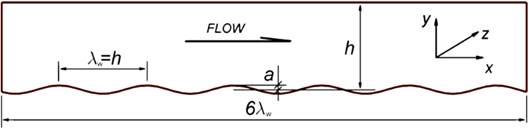Figure 1. Computational domain shape, for test case of 2a/λw=0.10. where the height h is measured from the middle of the sinusoidal bed. Non-dimensional, y+, measured from top of bed surface.

Table 1 gives all parameters of flow and mesh. The DNS parameters are the data obtained from Cherukat et al.  paper as sinusoidal bed with smooth bed surface in closed channel. While, MRM, is the experimental measurements results obtained by Hudson .

Table 1. Parameters for all test cases.

 Case Reb = ub h/υ L H W 2 a /λw sB 7250.0 6 λw λw 3λw ∞ W1 7250.0 6 λw λw 3λw 0.0100 W2 6300.0 6 λw λw 3λw 0.0375 W3 3800.0 6 λw λw 3λw 0.1000 DNS 3460.0 4 λw λw 2λw 0.1000 MRM 3380.0 4 λw λw 2λw 0.1000

In which, Reb is Reynolds number based on the bulk velocity

Table 2 contains all important parameters that considered in all tests. The wall friction velocity U*fit shows the least values with the increase with wave amplitude λw. It was observed from data processing.

Table 2. Cell dimensions and wall friction velocity values.

 Case Δx+ Δy+ Δz+ U*fit k+ SB 39.0 0.50-20.0 19.00 0.01370 0.00 W1 39.0 0.50-20.0 19.00 0.01370 4.00 W2 39.0 0.50-20.0 19.00 0.01270 15.0 W3 39.0 0.50-20.0 19.00 0.01220 40.0 DNS - - - - 40.0 MRM - - - - 40.0

In which, Δx+ = cell- non-dimensional x-direction, Δy+ = cell- non-dimensional y-direction and Δz+ = cell - non-dimensional z-direction. where, + refers to nondiemsionlized quantity.

Zero flux conditions are assumed as boundary assumptions for used sediment equation for the top and the bed surface.

Data sampling positions are presented in Figure 2.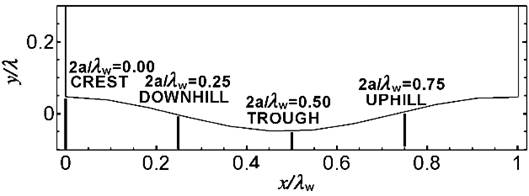Figure 2. Positions of data sampling.

3. Results of Flow

Time averaged and space averaged results are exhibited in this part. The period of time averaging is 30 h / ub but the space averaging must be in directions of span-wise (y) and stream-wise (x) called (SSA) or in the only direction of span-wise called (SA). The description of stream-wise lines is parallel to the gridlines in the channel flow and parallel to the sinusoidal bed shape near the bed.

From the survey in the literature, it is found only the results of DNS by Cherukat et al.  and the experimental measurements by Hudson , called MRM, to compare with these results. Cherukat et al. and Hudson show the flow separation point location and corresponding to the case W3 for this research.

The calculated shear stress (averaged) through wall at the bottom surface, average calculated bed friction (SA), is given in Figure 3 for test case W3. From this figure, it is found that the separation points and re-attachment points for at x /λw = 0.190 and 0.580.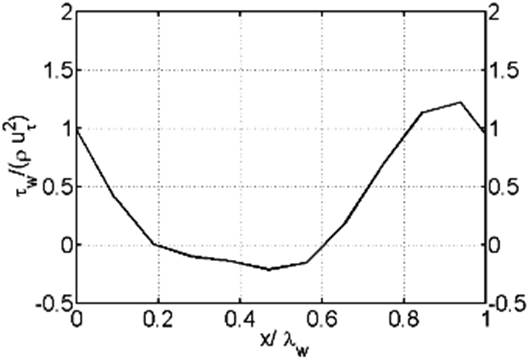Figure 3. Bed surface averaged wall shear stresses, Case (W3) (Averaging SA).

Table 3 gives a comparison between the obtained separation and reattachment point results with the corresponding ones given by Cherukat et al.  and the experimental results of Hudson .

Table 3. Locations of separation and reattachment point for DNS and MRM.

 Point of Separation Point of Reattachment W3 x /λw = 0.190 x /λw = 0.580 DNS x /λw = 0.140 x /λw = 0.590 MRM x /λw = 0.220 x /λw = 0.580

From this table it is found that the all of W3, DNS and MRM gave approximately the same results for the reattachment point. The experimental obtained results (MRM) agree with the present obtained results by LES.

A comparison between the average velocities in the direction of streamwise between the obtained solution by LES and DNS results of Cherukat et al.  is illustrated in Figure 4.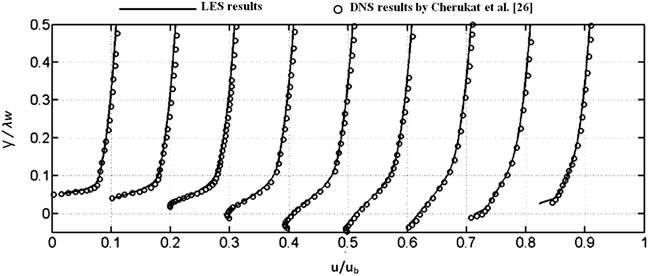Figure 4. Mean velocity profiles for LES and DNS in the direction of streamwise at different locations for x/λw ranges from 0.1 to 0.8.

The logarithmic law for rough bed could be applied for calculating velocity as non-dimensionalized and compared with the corresponding velocity results obtained by LES simulation. A single profile of velocity for each case is shown and all the results are averaged values. The logarithmic profile reference - modified for rough bed - obtained from the following equation: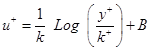(1)

In which, u+ = u /u*, y+= y × u*/ υ, k+ = ks × u* /υ where, ks = wall roughness height. The logarithmic law coefficient B equals 11.5 for W2 and 8.5 for W3, these values of B were given by the approximation of best fit. Value of B for W1 case could be neglected as there is very small difference between smooth bed and W1 case of rough bed. The standard logarithmic law is used to compare with smooth bed as: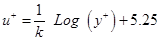(2)

Figure 5a gives the non-dimensionalized stream and spanwise velocity profiles, Fig. (5b, c and d) exhibit the profiles of velocity at different locations along the bed wall at W1, W2 and W3 cases respectively. All results in these figures are averaged in time and in spanwise direction. It is appeared that in W3 case, the profile of velocity near the wall at y+ >100 is parallel compared with the smooth bed case. This result is also the same one shown by the solutions given by Kuzan et al. , Nakagawa and Hanratty  and Nakagawa et al. . Also, it is shown that at the zone above y+ = 100 the velocity profile is logarithmic profile and not affected by the location along wall.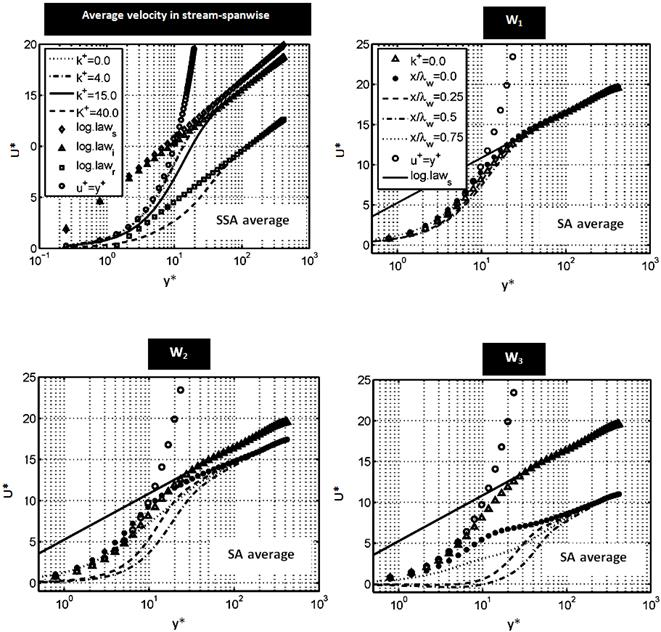Figure 5. Average velocity profiles compared with the log-laws in case of k+= 4, log-lawi in case of k+=15 and log-lawT in case of k+=40.

4. Modeling of Sediment Transport

This part includes the results of sediment concentration and a detailed comparison between the results obtained from LES and Rouse profile. Figure 6 gives the distribution of different volumetric fraction of concentrations of sediment rates by LES and the theoretical profile of Rouse. While the equation used for calculating the Rouse profile separately is:(3)

In which, qs = Sediment transport concentration, ya= Reference height = 0.5y/λw, qa = concentrations of sediment-reference. Where ya = h/2 (called reference height) then the parameter Z could be calculated by (υs/k u* β) in which k = Von-Karman coefficient and β = 1.4, where υs = settling velocity.

From this figure, it is noticed that the sediment transport concentrations in W1 and W2 is approximately the case of smooth bed but in W3 case, there is a noticeable difference appeared.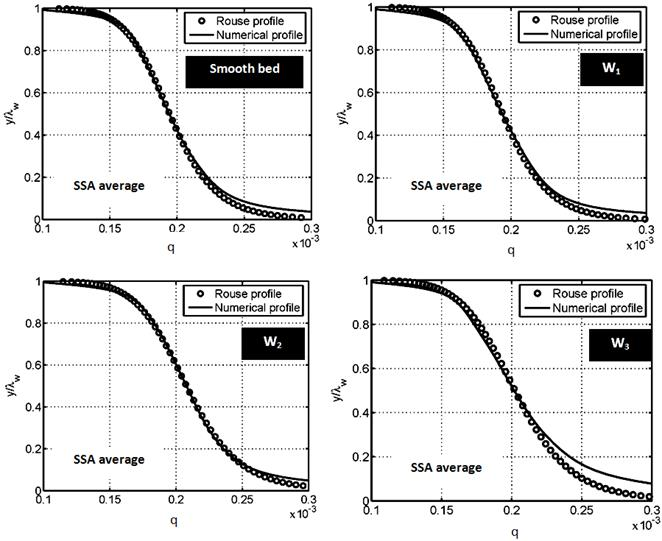Figure 6. Concentration of sediment transport.

It is shown from the comparison profiles that the deviations occur mainly near the bed. This appeared clearly in case W3 at the higher concentrations of sediments in the lower part. At points of x/λw = 0.20 and 0.58 in W3 case, a region of full reversed flow behind the bed crest and higher levels of velocity fluctuations occur and causes a higher sediment transport rates. The fluctuations of velocity behind the crest of bed wave, mainly for zone located at 0.0 < x/λw < 1.0 is given in Figure 7.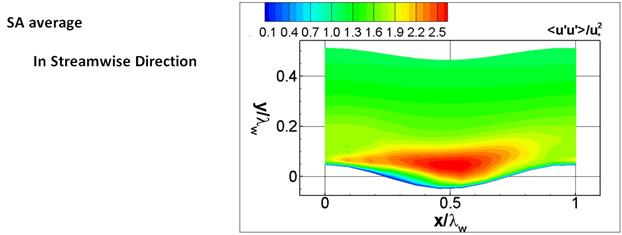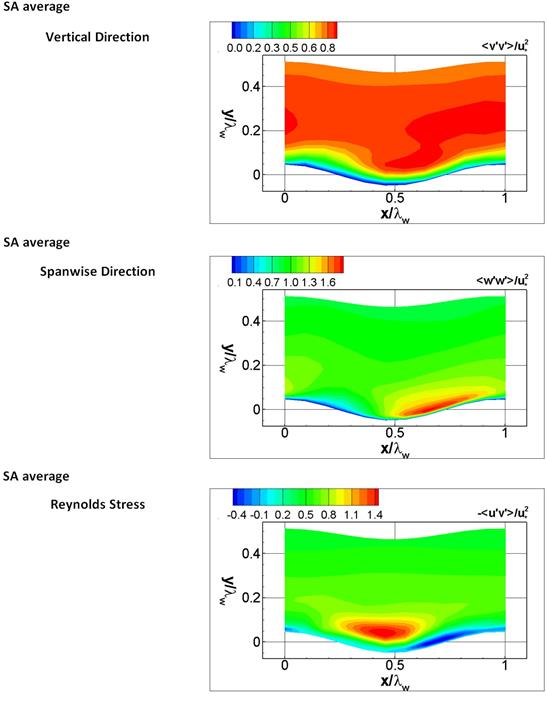Figure 7. Obtained fluctuation of velocity in W3 case.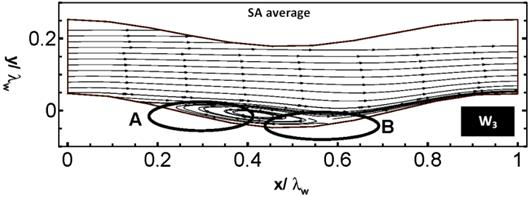Figure 8. Sketch of isolines of velocity for (a) Settling of sediment particles zone (b) Zone of recirculation (removing zone), W3 case.

At the existence of zones of flow recirculation, the sediment concentration increases with the lower velocities and the level of turbulence more than the other regions along the bed sinusoidal shape. These zones could be described as a sediment trap, as shown in Figure 8 and Figure 9.

In region A, the recirculation zone of eddies and stagnation flow with, but region B the sediment moves a way. Figure 9 shows qualitative images of the concentrations of sediment near the bed and scaled turbulent kinetic energy calculated by the friction velocity.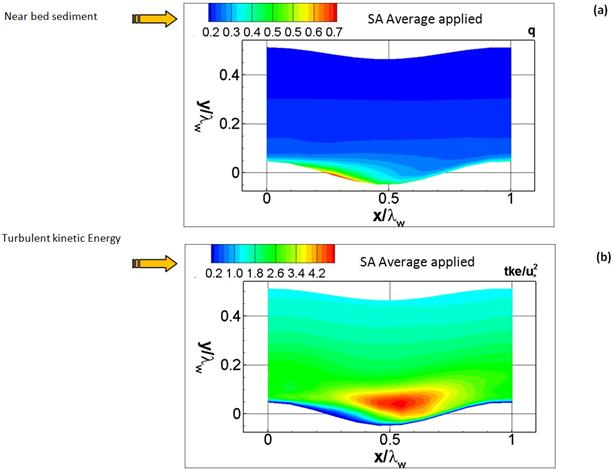Figure 9. Qualitative sediment transport near the bed.

It could be appeared that the sediment transport concentrations increases in the regions of low turbulent kinetic energy levels, but in high levels of turbulence kinetic energy, the concentrations of sediments is small.

5. Conclusions

In research, the sensitivity of the sediment transport and of the flow passes through rough bed channels is studied. Four scenarios of bed cases are selected for testing. First test case, using large eddy simulation, LES, an investigation of smooth bed is performed for ReT equals 395 (number of Reynolds calculated using the mean friction velocities at wall). The other three corrugated sinusoidal bed shape cases are assumed with different amplitudes and constant wavelengths An eddy viscosity model that is adapted to wall is applied in this research; while the subgrid scale quantity is depend on the hypothesis gradient. A comparison between the data of Cherukat et al.  obtained from Direct Numerical Simulation (DNS) and the data measured by Hudson et al.  with the corresponding computed flow is done. It was concluded that the obtained numerical gives a good agreement with the profile deduced by Rouse equation through the zone above the near wall layer. At the near wall zone, there is a clear difference between the numerical solution using LES and Rouse profile. Generally, it is clearly found that the bed shape and the fluctuation of flow velocities have a great effect on the sediment transportation concentrations but only in the near bed zone.

References

1. Ashrafian A., Andersson H. and Manhart M. "DNS of turbulent ﬂow in a rod-roughened channel", International Journal of Heat and Fluid Flow, Vol. 25, pp. 373–383, 2004.
2. Bhaganagar K., Kim J. and Coleman G. "Effects of wall bounded roughness on turbulence", Int. J. of Heat and Fluid Flow, Vol. 72, pp. 463–492, 2004.
3. Boersma W., Laven J. and Stein H. "Computer simulations of shear thickening of concentrated dispersions", J. of Rheology, Vol. 39, pp. 841–859, 1995.
4. Cellino M. "Experimental study of suspension ﬂow in open channels", PhD thesis, Ec. Poly. Fed. de Lau., Lausanne, Switz, 1998.
5. Cherukat P., Na Y., Hanratty T. and Mclughlin J. "Direct numerical simulation of a fully developed turbulent ﬂow over a wavy wall", Theoretical and Computational Fluid Dynamics, Vol. 11, pp. 109 –134, 1998.
6. Choi H. and Suzuki K. "Large eddy simulation of turbulent ﬂow and heat transfer in a channel with one wavy wall, Int. J. of Heat and Fluid Flow, 2005.
7. Einstein H. and Barbarossa N. "River channel roughness", J. of Hydr. Eng., Vol. 117, 1952.
8. Graf W. and Cellino M. "Suspension ﬂows in open channels: experimental study", AHR J. of Hydr. Res., Vol. 40, No. 4, pp. 435–447, 2002.
9. Grass J. "Structural features of turbulent ﬂow over smooth and rough boundaries", Journal of Fluid Mechanics, Vol. 50, pp. 233–255, 1971.
10. Heredia M. "Numerical modeling of turbulent ﬂow over rough surfaces", PhD thesis, Dept. of Bur. Bouwk. Lab. Vo. Hydr., Kath. Univ. Leuv. Leuven, Belgium, 2009.
11. Hudson J., Dykhno L. and Hanratty T. "Turbulence production in ﬂow over a wavy wall", Experiments in Fluids, Vol. 20, pp. 256– 265,1996.
12. Jimenez J. "Turbulent ﬂows over rough walls", Annual Review of Fluid Mechanics, Vol. 36, pp. 173–196, 2004.
13. Kuzan J., Adrian R. and Hanratty T. "Turbulence ﬂows with incipient separation over solid waves", Exper. in Fluids, Vol. 7, pp. 88–98, 1989.
14. Lee C. "Large eddy simulation of rough-wall turbulent boundary layers", Amer. Inst. of Aero. & Astron., Vol. 40, pp. 2127–2130, 2002.
15. Lely M. "Hydraulics roughness in sediment laden ﬂow".
16. Leonardi S., Orlandi P., Smalley R., Djenidl L. and An- Tonia R. "Direct numerical simulations of turbulent ﬂow with transverse square bars on one wall", J. of Flu. Mech., Vol. 491, pp. 229–238, 2003.
17. Miyake Y., Tsujimoto K. and Nagal N. "Numerical simulation of channel ﬂow with a rib-roughened wall", Journal of Turbulence, Vol. 035, 2002.
18. Murray P. "Settling velocities and vertical diffusion of particles in turbulent water", J. of Sedi. Petr., Vol. 75, pp. 1647–1654, 1970.
19. Nakagawa S. and Hanratty T. "Inﬂuence of a wavy boundary on turbulence intermediate roughened and hydraulically smooth surface", Experiments in Fluids Vol. 35, pp. 437–447, 2003.
20. Nakagawa S., Na Y. and Hanratty T. "Inﬂuence of a wavy boundary on turbulence. i. highly rough surface", Exper. in Fluids, Vol. 35, pp. 422–436, 2003.
21. Nikuradse J. "Laws of ﬂow in rough pipes", Technical Memorandum, NACA-TM-1292, NASA, 1950.
22. Orlandi P., Leonardi S., Tuzi R. and Antonia R. "Direct numerical simulation of turbulent channel ﬂow with wall velocity disturbances", Phys. of Flui, Vol. 15, No. (12), 2003.
23. Orlandi P. and Leonardi S. "Passive scalar in a turbulent channel ﬂow with wall velocity disturbances", Flow & Turbulence and Combustion, Vol. 72, pp. 181–197, 2004.
24. Orlandi P. and Leonardi S., Djenidi L. and Antonia R. "Structure of turbulent channel ﬂow with square bars on one wall", International Journal of Heat and Fluid Flow, Vol. 25, pp. 384–392, 2004.
25. Stoesser T., Mathiey F., Frohlich J. and Rodi W. "LES of ﬂow over multiple cubes", Ercoftac Bull. March, 2003.
26. Tseng Y. and Ferziger J. "Large eddy simulation of turbulent wavy boundary ﬂow - illustration of vortex dynamics", J. of Turb, 2004.
27. Yoon J. and Kang S. "A numerical model of sediment-laden turbulent ﬂow in an open channel", Canadian J. of Civil Eng., Vol. 32, pp. 233–240, 2005.

 Contents 1. 2. 3. 4. 5.
Article ToolsAbstractPDF(1244K)